The SIMILARITY Procedure

Time Series Transformation

Transformations are useful when you want to stabilize the time series before computing the similarity measures. There are four transformations available, for strictly positive series only. Let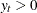be the original time series, and let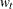be the transformed series. The transformations are defined as follows:

Log

is the logarithmic transformation,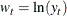Logistic

is the logistic transformation,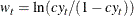where the scaling factoris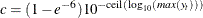and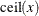is the smallest integer greater than or equal to x.

Square root

is the square root transformation,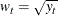Box-Cox

is the Box-Cox transformation,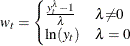User-Defined

is the transformation computed by a user-defined subroutine that is created by using the FCMP procedure, where User-Defined is the subroutine name.

Other time series transformations can be performed prior to invoking the SIMILARITY procedure by using the SAS/ETS EXPAND procedure or the DATA step.